Monday, January 20, 2020
Home > Latest Announcement > NMTC 2018 Question Papers With Solutions Primary Level

# NMTC 2018 Question Papers With Solutions Primary Level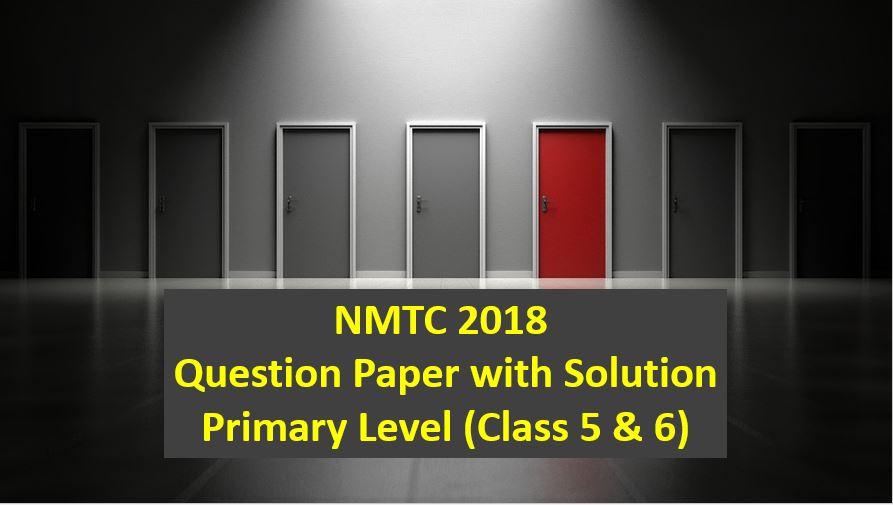# NMTC 2018 Question Papers with Solutions Primary Level

NMTC is an acronym for National Mathematics Talent Contest. NMTC is conducted by AMTI (Association of Mathematics Teachers of India) to identify the talent of the students in Mathematics. In this post, NMTC 2018 Question Papers with Solutions Primary Level is published.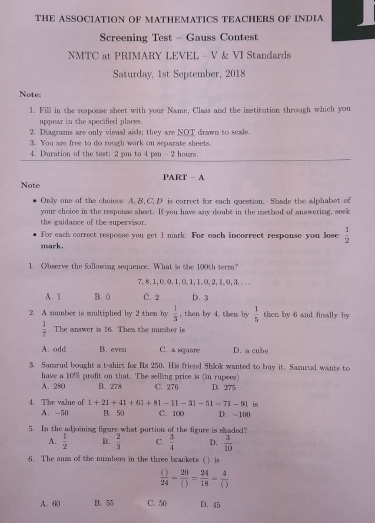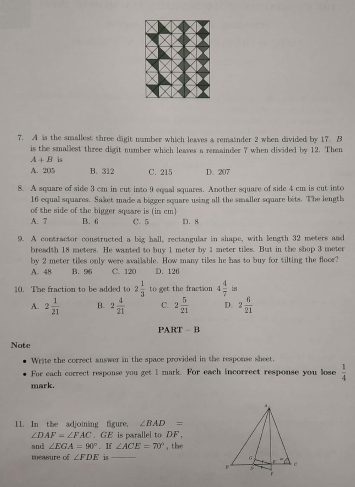Part A: Instruction:

Only One of the choices A, B, C, D is correct. For each correct response, you get 1 mark. For each incorrect response, you lose 1/2 mark. NMTC 2018 Question Papers with Solutions Primary Level.

NMTC 2018 Question Papers With Solutions Primary Level Ques No 1:

Observe the following sequence. What is the 100th term? 7, 8, 1, 0, 0, 1, 0, 1, 1, 0, 2, 1, 0, 3, …

Options:

A. 1

B. 0

C. 2

D. 3

Solution:NMTC 2018 Question Papers With Solutions Primary Level Ques No 2

A number is multiplied by 3 then by 1/3, then by 4, then by 1/5 then by 6 and finally by 1/7. The answer is 16. Then the number is

Options:

A. Odd

B. Even

C. A square

D. A Cube

Solution: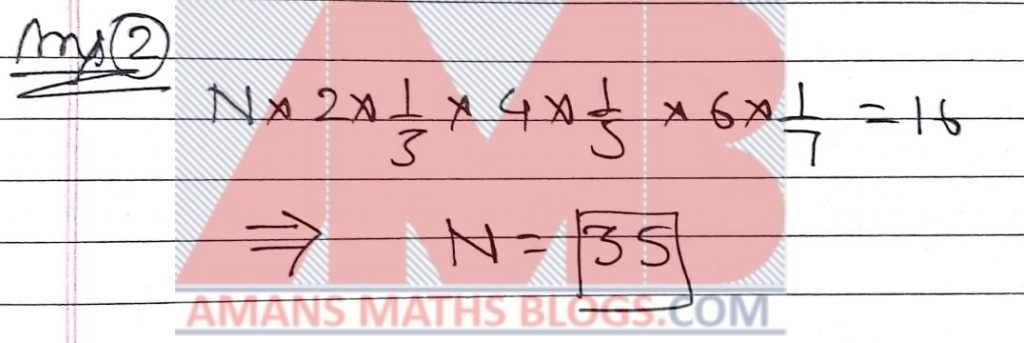NMTC 2018 Question Papers With Solutions Primary Level Ques No 3

Samrud bought a t-shirt for Rs 250. His friend Shlok wanted to buy it. Samrud wants to have 10% profit on that. The selling price is (in rupees)

Options:

A. 280

B. 278

C. 276

D. 275

Solution:NMTC 2018 Question Papers With Solutions Primary Level Ques No 4

The value of 1 + 21 + 41 + 61 + 81 – 11 – 31 – 51 – 71 – 91 is

Options:

A. -50

B. 50

C. 100

D. -100

Solution:NMTC 2018 Question Papers With Solutions Primary Level Ques No 5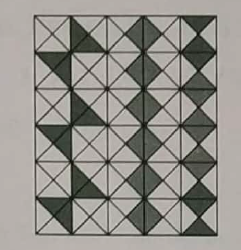Options:

A. 1/2

B. 2/3

C. 3/4

D. 3/10

Solution: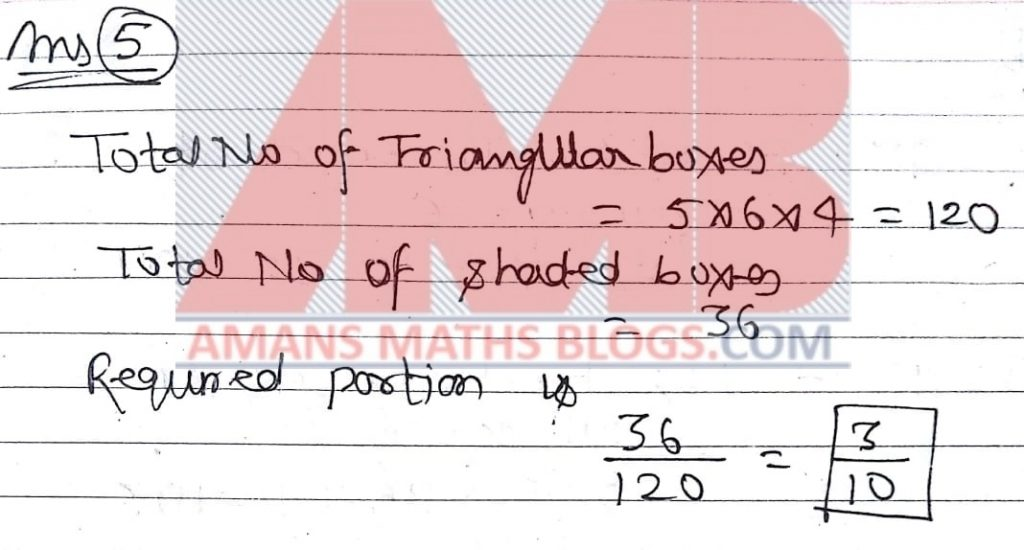NMTC 2018 Question Papers With Solutions Primary Level Ques No 6

The sum of the numbers in the three brackets () is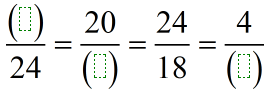Options:

A. 60

B. 55

C. 50

D. 45

Solution: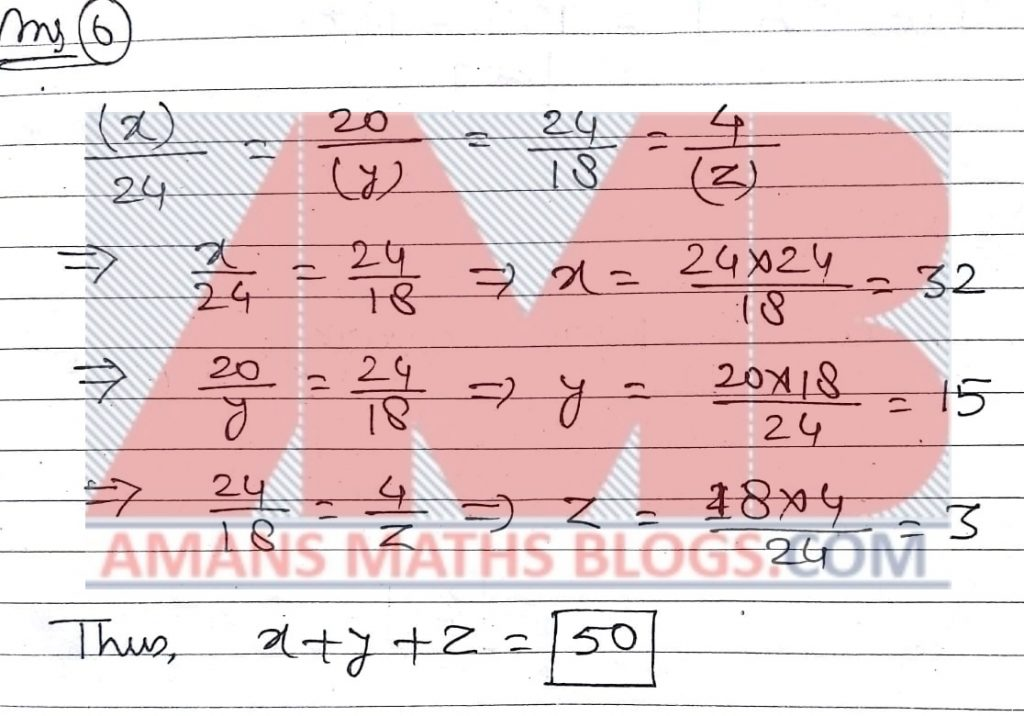NMTC 2018 Question Papers With Solutions Primary Level Ques No 7

A is the smallest three-digit number which leaves a remainder 2 when divided by 17. B is the smallest three-digit number which leaves a remainder 7 when divided by 12. Then A + B is

Options:

A. 205

B. 312

C. 215

D. 207

Solution:NNMTC 2018 Question Papers With Solutions Primary Level Ques No 8

A square of side 3 cm in cut into 9 equal squares. Another square of side 4 cm is cut into 16 equal squares. Saket made a bigger square using all the smaller square bits. The length of the side of the bigger square is (in cm)

Options:

A. 7

B. 6

C. 5

D. 8

Solution: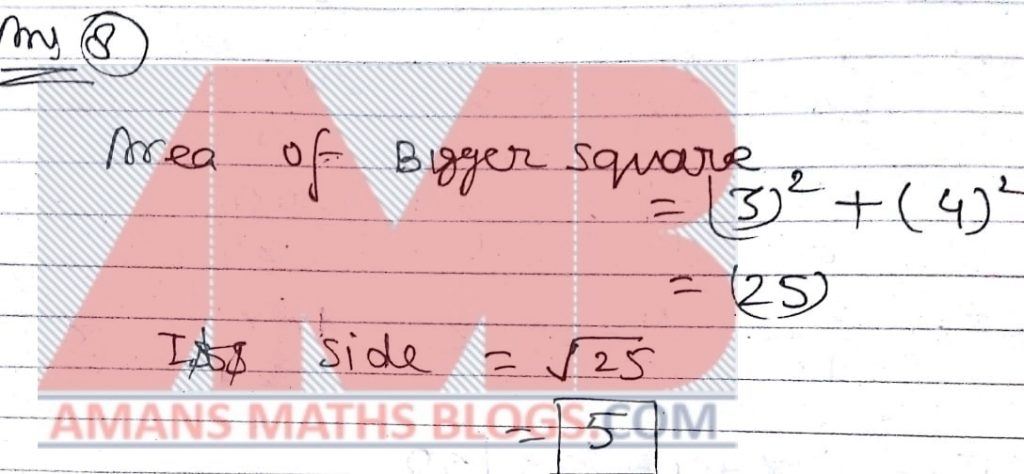NMTC 2018 Question Papers With Solutions Primary Level Ques No 9

A contractor constructed a big hall, rectangular in shape, with length 32 meters and breadth 18 meters. He wanted to buy 1 meter by tiles. But in the shop 3 meter by 2 meter tiles only were available. How many tiles he has to buy for tilting the floor?

Options:

A. 48

B. 96

C. 120

D. 126

Solution: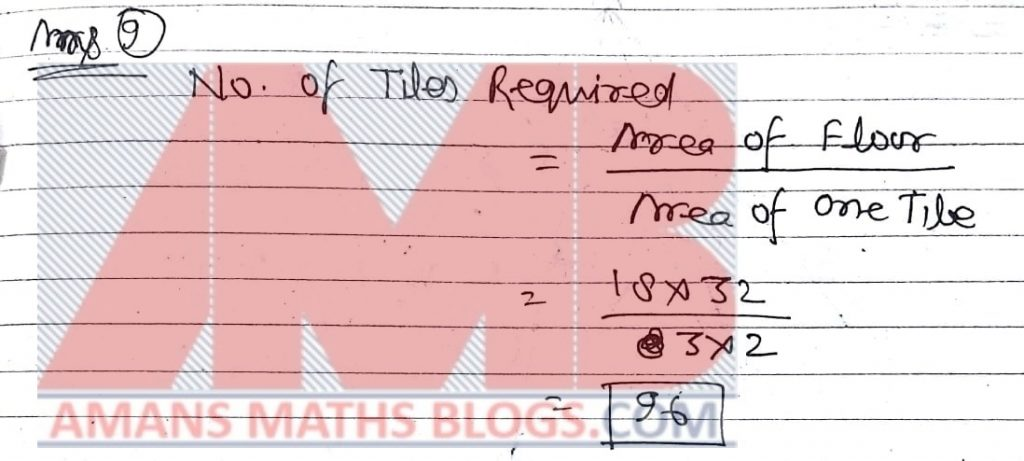NMTC 2018 Question Paper With Solutions Primary Level Ques No 10

The fraction to be added toto get the fractionis

Options:

A.B.C.D.Solution: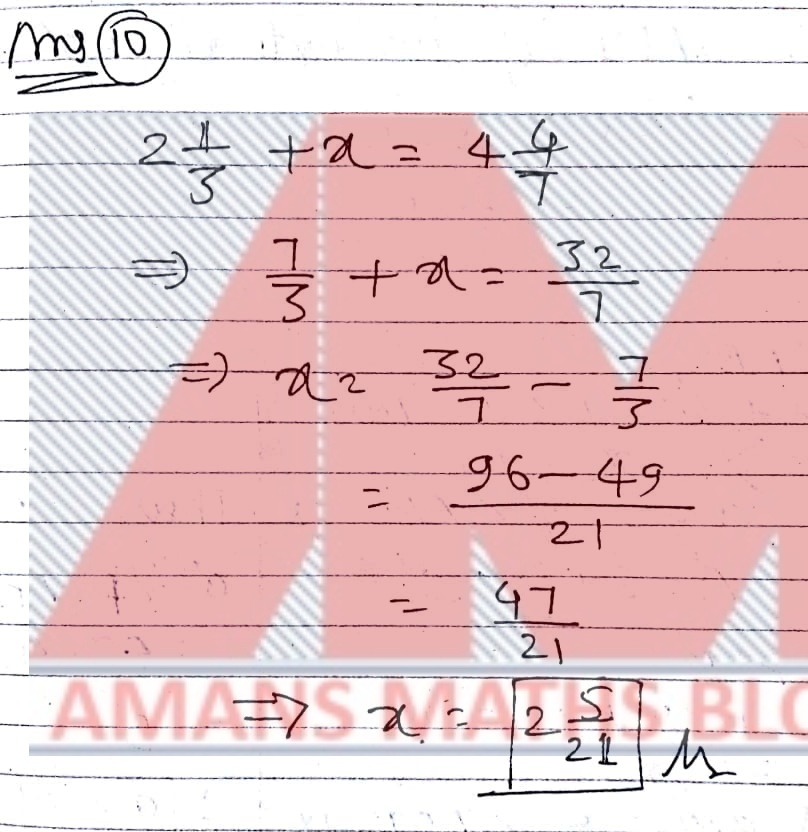Part B: Instruction:

Write the correct answer in the space provided in the responsive sheet. For each correct response, you get 1 mark. For each incorrect response, you lose 1/4 mark.

NMTC 2018 Question Paper With Solutions Primary Level Ques No 11

In the adjoining figure, angle BAD = angle DAF = angle FAC. GE is parallel to DF, and angle EGA = 90 Degree. If angle ACE = 70 Degree, the measure of the angle FDE is________.Solution: BONUS as some information is missing in the question.

NMTC 2018 Question Paper With Solutions Primary Level Ques No 12

ABC is a triangle in which the angles are in the ratio 3 : 4 : 5. PQR is a triangle in which the angles are in the ratio 5 : 6: 7. The difference between the least angle of ABC and the least angle of PQR is a Degree (alpha). Then a (alpha)______

Solution:NMTC 2018 Question Paper With Solutions Primary Level Ques No 13

Samrud had to multiply a number by 35. By mistake he multiplied by 53 and got a result 720 more. The new product is_______

Solution: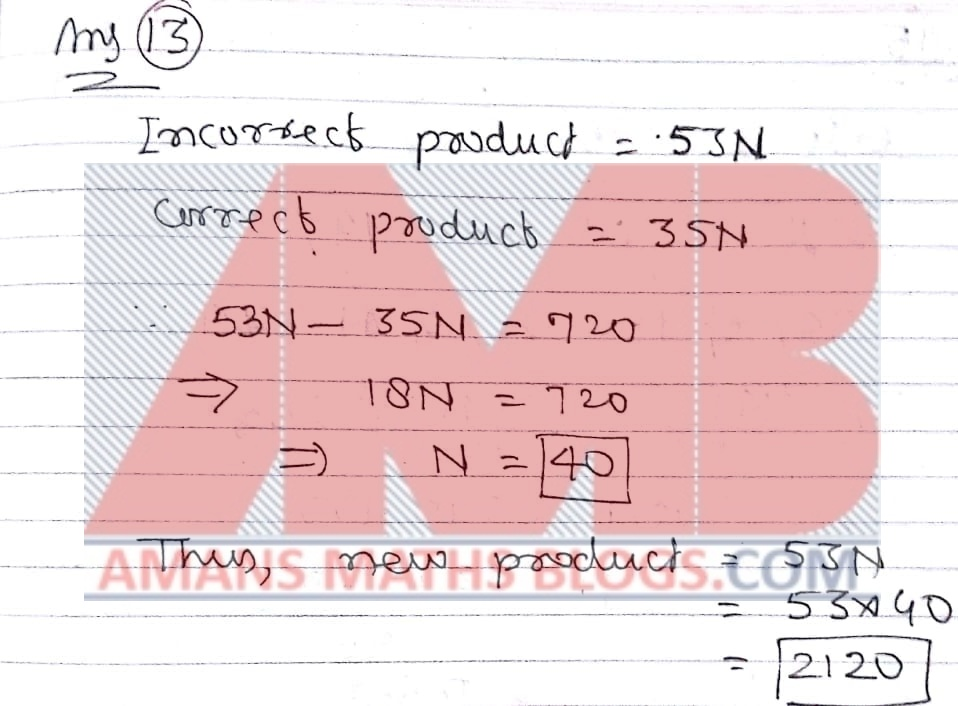NMTC 2018 Question Paper With Solutions Primary Level Ques No 14

Vishva plays football every 4th day. He played on a Tuesday. He plays football on a Tuesday again in _____ days.

Solution:NMTC 2018 Question Paper With Solutions Primary Level Ques No 15

In an elementary school 26% of the students are girls. If there are 240 less girl than boys, then the strength of the school is_____

Solution:NMTC 2018 Question Paper With Solutions Primary Level Ques No 16

There are three concentric circles as shown in the figure. The radii of them are 2 cm, 4 cm and 6 cm. The ratio of the area of the shaded region to the area of the dotted region is a/b where a, b are integers and have no common factors other than 1. Then a + b = ______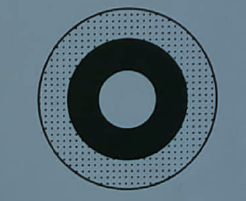Solution: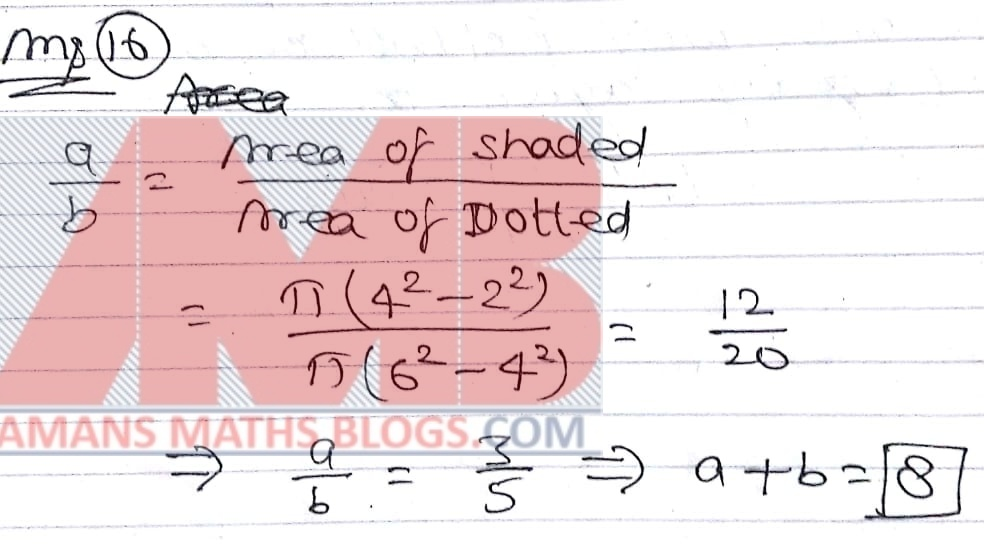NMTC 2018 Question Paper With Solutions Primary Level Ques No 17

In The value ofis ______.

Solution: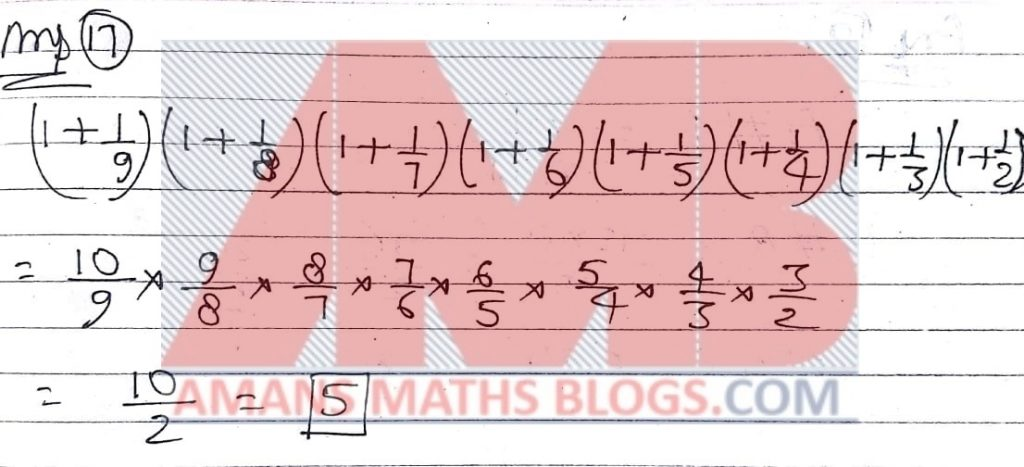NMTC 2018 Question Paper With Solutions Primary Level Ques No 18

When a two digit number divides 265, the remainder is 5. The number of such two digit numbers is______

Solution:NMTC 2018 Question Paper With Solutions Primary Level Ques No 19

If A#B = (AB)/(A+B), the value of (12#8)/(8#4) + (10#6)/(6#2) is______

Solution:NMTC 2018 Question Paper With Solutions Primary Level Ques No 20

When water becomes ice, its volume increases by 10%. When ice melts into water its volume decrease by a%. Then a = ­­­________

Solution:Get URL of this EBook on Facebook Page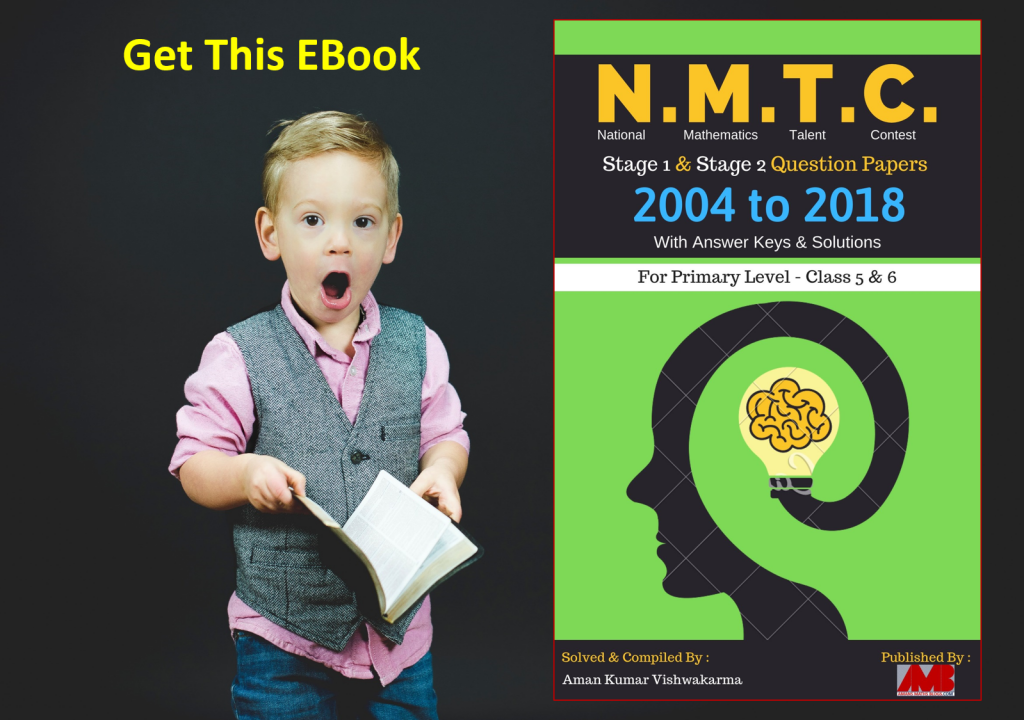Click On Image To Get EBOOK

error: Content is protected !!